# 1 Chapter 3 Topics Announcements All homework quizzes

• Slides: 181 Chapter 3 Topics • Announcements – All homework quizzes completed on line – Extension for chapter 2 until Jan 28, 8 pm to allow for minor computer issues to be resolved – Monday is a review day • Topics – Using percent composition » Emperical and molecular formulas – Calculating a formula – Formula of a compound from combining masses – Formula from mass data – Hydrated compounds Kull Spring 2007 Chem 105 Lsn 5 © 2006 Brooks/Cole - Thomson 1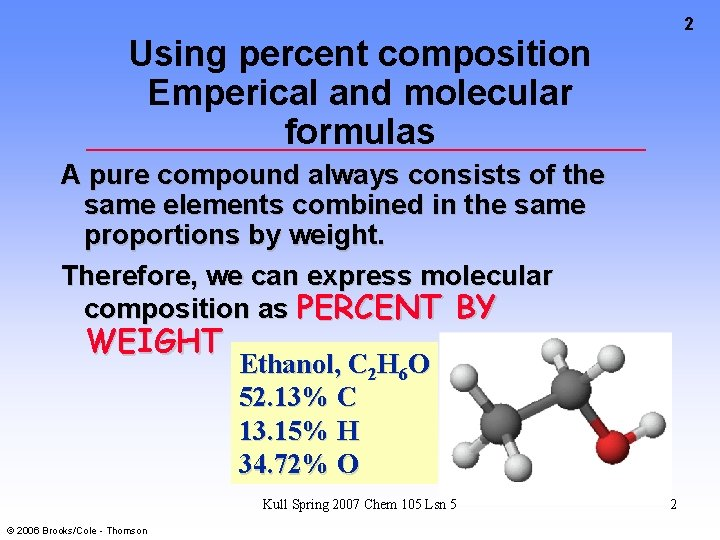2 Using percent composition Emperical and molecular formulas A pure compound always consists of the same elements combined in the same proportions by weight. Therefore, we can express molecular composition as PERCENT BY WEIGHT Ethanol, C 2 H 6 O 52. 13% C 13. 15% H 34. 72% O Kull Spring 2007 Chem 105 Lsn 5 © 2006 Brooks/Cole - Thomson 23 Percent Composition Consider NO 2, Molar mass = ? What is the weight percent of N and of O? What are the weight percentages of N and O in NO? Kull Spring 2007 Chem 105 Lsn 5 © 2006 Brooks/Cole - Thomson 34 Calculating a formula In chemical analysis we determine the % by weight of each element in a given amount of pure compound and derive the EMPIRICAL or SIMPLEST formula. PROBLEM: A compound of B and H is 81. 10% B. What is its empirical formula? Kull Spring 2007 Chem 105 Lsn 5 © 2006 Brooks/Cole - Thomson 45 A compound of B and H is 81. 10% B. What is its empirical formula? • Because it contains only B and H, it must contain 18. 90% H. • In 100. 0 g of the compound there are 81. 10 g of B and 18. 90 g of H. • Calculate the number of moles of each constitutent. Kull Spring 2007 Chem 105 Lsn 5 © 2006 Brooks/Cole - Thomson 56 A compound of B and H is 81. 10% B. What is its empirical formula? Calculate the number of moles of each element in 100. 0 g of sample. Kull Spring 2007 Chem 105 Lsn 5 © 2006 Brooks/Cole - Thomson 6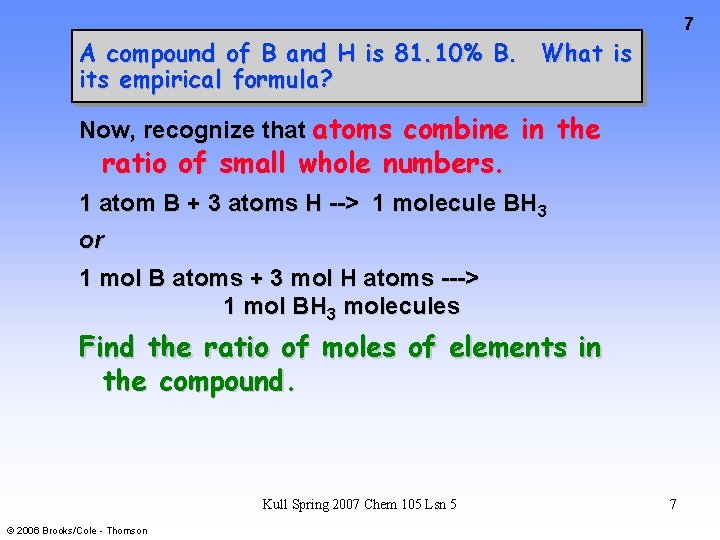7 A compound of B and H is 81. 10% B. What is its empirical formula? Now, recognize that atoms combine in the ratio of small whole numbers. 1 atom B + 3 atoms H --> 1 molecule BH 3 or 1 mol B atoms + 3 mol H atoms ---> 1 mol BH 3 molecules Find the ratio of moles of elements in the compound. Kull Spring 2007 Chem 105 Lsn 5 © 2006 Brooks/Cole - Thomson 7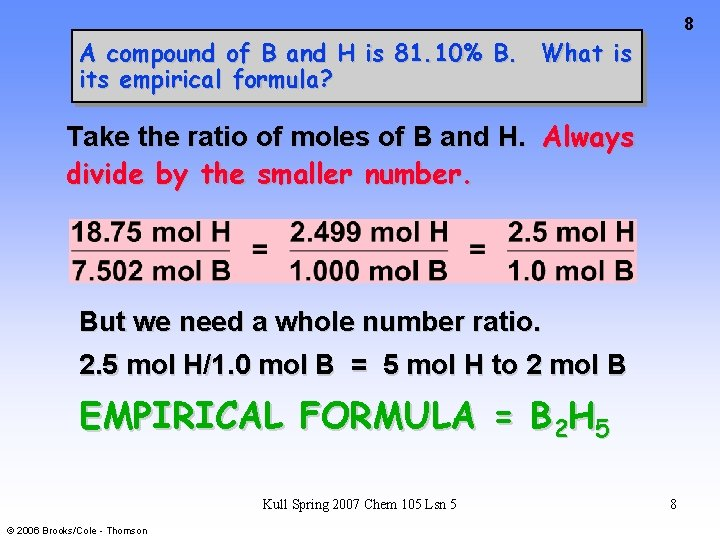8 A compound of B and H is 81. 10% B. What is its empirical formula? Take the ratio of moles of B and H. Always divide by the smaller number. But we need a whole number ratio. 2. 5 mol H/1. 0 mol B = 5 mol H to 2 mol B EMPIRICAL FORMULA = B 2 H 5 Kull Spring 2007 Chem 105 Lsn 5 © 2006 Brooks/Cole - Thomson 8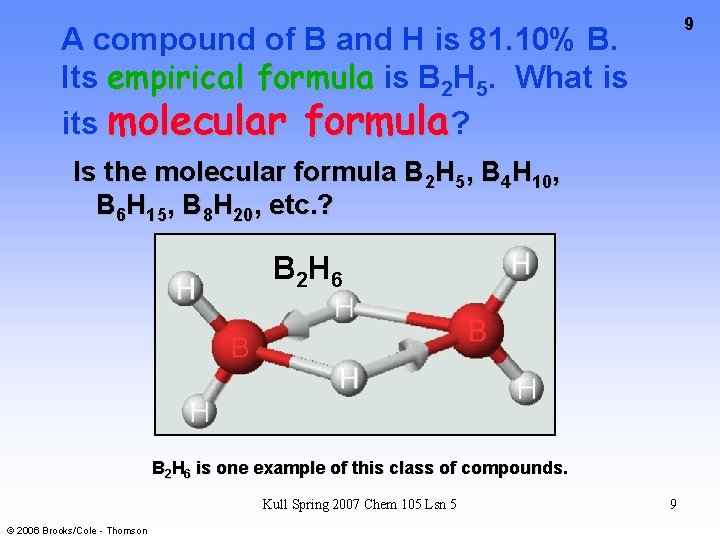9 A compound of B and H is 81. 10% B. Its empirical formula is B 2 H 5. What is its molecular formula? Is the molecular formula B 2 H 5, B 4 H 10, B 6 H 15, B 8 H 20, etc. ? B 2 H 6 is one example of this class of compounds. Kull Spring 2007 Chem 105 Lsn 5 © 2006 Brooks/Cole - Thomson 910 A compound of B and H is 81. 10% B. Its empirical formula is B 2 H 5. What is its molecular formula? We need to do an EXPERIMENT to find the MOLAR MASS. Here experiment gives 53. 3 g/mol Compare with the mass of B 2 H 5 = 26. 66 g/unit Find the ratio of these masses. Molecular formula = B 4 H 10 Kull Spring 2007 Chem 105 Lsn 5 © 2006 Brooks/Cole - Thomson 10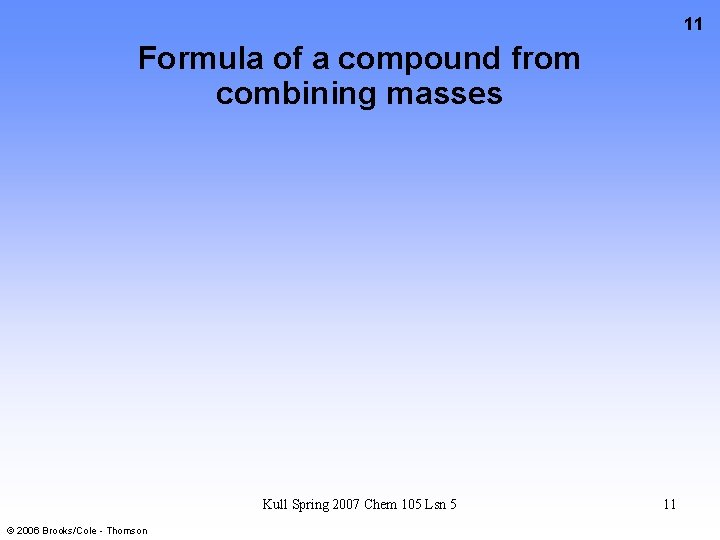11 Formula of a compound from combining masses Kull Spring 2007 Chem 105 Lsn 5 © 2006 Brooks/Cole - Thomson 1112 Formula of a compound from combining masses DETERMINE THE FORMULA OF A COMPOUND OF Sn AND I Sn(s) + some I 2(s) ---> Sn. Ix 4+ , 2 + © 2006 Brooks/Cole - Thomson 1 - Kull Spring 2007 Chem 105 Lsn 5 1213 Data to Determine the formula of a Sn—I Compound • Reaction of Sn and I 2 is done using excess Sn. • Mass of Sn in the beginning = 1. 056 g • Mass of Sn remaining (recovered) = 0. 601 g • Mass of iodine (I 2) used = 1. 947 g (See p. 125) Convert these masses to moles Kull Spring 2007 Chem 105 Lsn 5 © 2006 Brooks/Cole - Thomson 13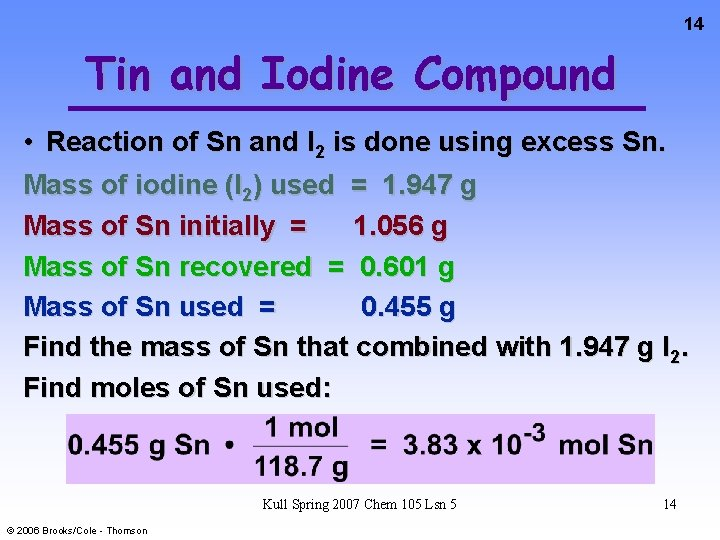14 Tin and Iodine Compound • Reaction of Sn and I 2 is done using excess Sn. Mass of iodine (I 2) used = 1. 947 g Mass of Sn initially = 1. 056 g Mass of Sn recovered = 0. 601 g Mass of Sn used = 0. 455 g Find the mass of Sn that combined with 1. 947 g I 2. Find moles of Sn used: Kull Spring 2007 Chem 105 Lsn 5 © 2006 Brooks/Cole - Thomson 14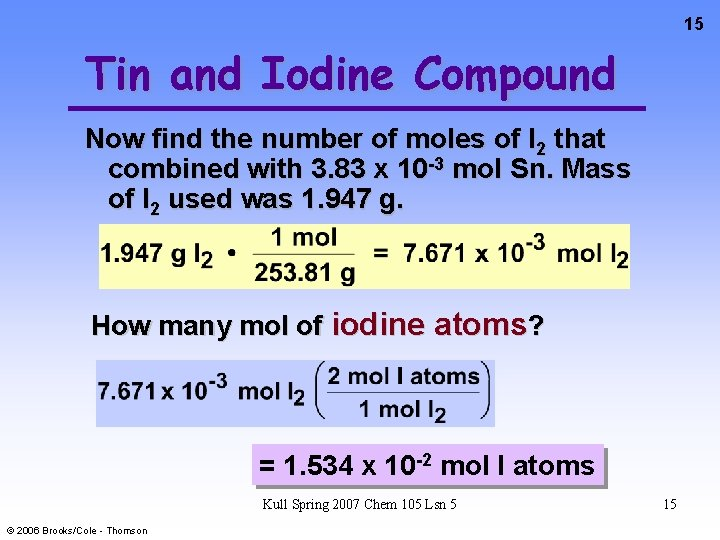15 Tin and Iodine Compound Now find the number of moles of I 2 that combined with 3. 83 x 10 -3 mol Sn. Mass of I 2 used was 1. 947 g. How many mol of iodine atoms? = 1. 534 x 10 -2 mol I atoms Kull Spring 2007 Chem 105 Lsn 5 © 2006 Brooks/Cole - Thomson 15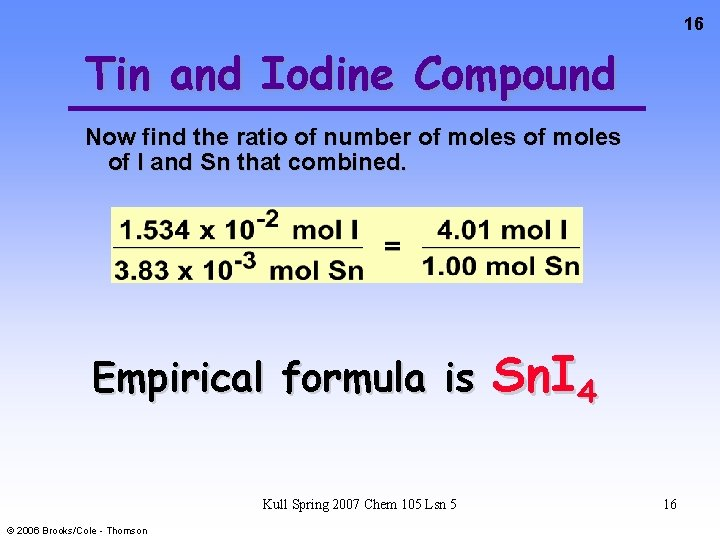16 Tin and Iodine Compound Now find the ratio of number of moles of I and Sn that combined. Empirical formula is Kull Spring 2007 Chem 105 Lsn 5 © 2006 Brooks/Cole - Thomson Sn. I 4 1617 Formula from mass data Kull Spring 2007 Chem 105 Lsn 5 © 2006 Brooks/Cole - Thomson 1718 Hydrated compounds Kull Spring 2007 Chem 105 Lsn 5 © 2006 Brooks/Cole - Thomson 18